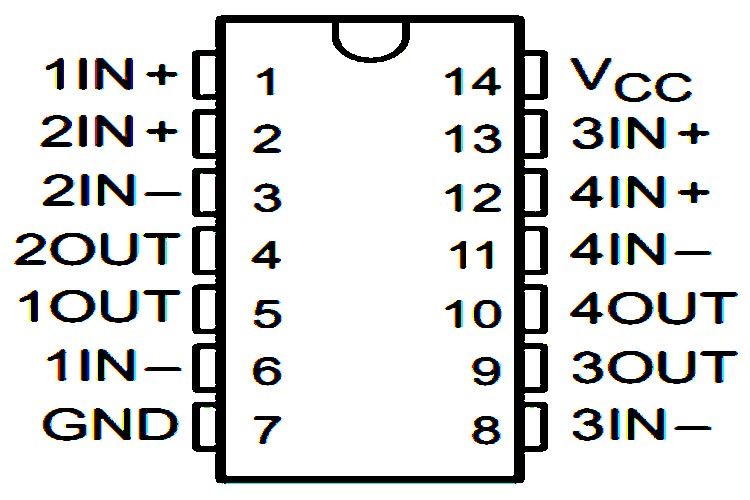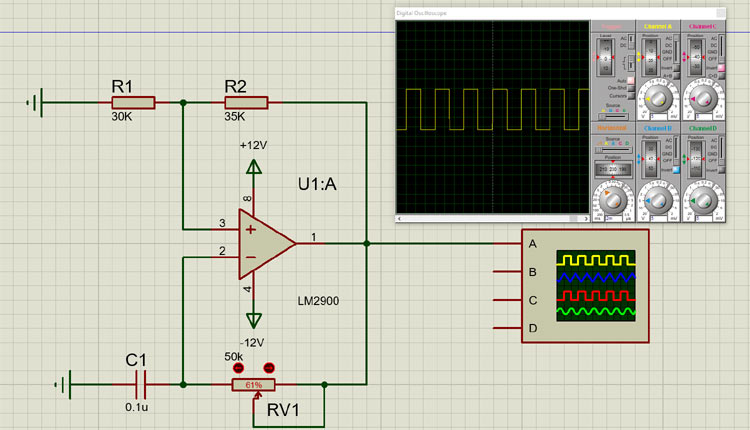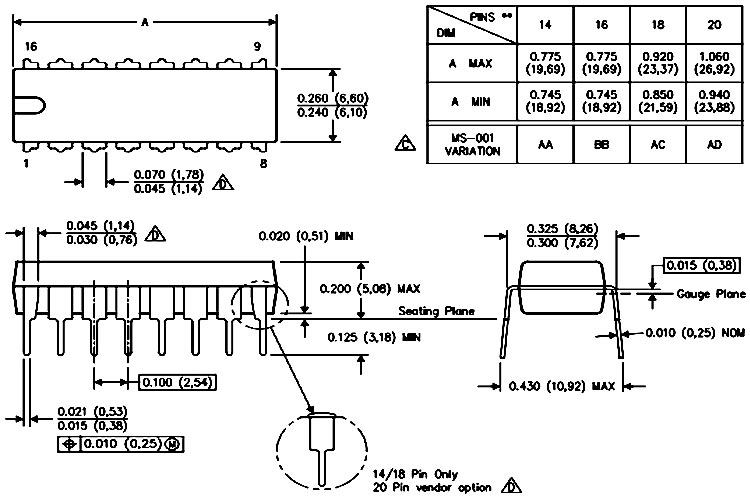# LM2900 Quadruple Norton Operational Amplifier

The LMX900 series has two versions: the LM2900 is characterized for operation from –40°C to 85°C, and the LM3900 is characterized for operation from 0°C to 70°C. These devices consist of four independent, high gain frequency-compensated Norton operational amplifiers that were designed specifically to operate from a single supply over a wide range of voltages. Operation from split supplies is also possible. The low supply current drain is essentially independent of the magnitude of the supply voltage. These devices provide wide bandwidth and large output voltage swings.

### LM2900 Op-Amp Pinout Configuration

 Pin Number Pin Name Description 1 1 IN+ Non-Inverting Input of Op-Amp 1 2 2 IN+ Non-Inverting Input of Op-Amp 2 3 2 IN- Inverting Input of Op-Amp 2 4 2 Output Output of Op-Amp 2 5 1 Output Output of Op-Amp 1 6 1 IN- Inverting Input of Op-Amp 1 7 GND Ground of Supply Voltage 8 3IN- Inverting Input of Op-Amp 3 9 3 OUT Output of Op-Amp 3 10 4 OUT Output of Op-Amp 4 11 4 IN- Inverting Input of Op-Amp 4 12 4 IN+ Non-Inverting Input of Op-Amp 4 13 3 IN+ Non-Inverting Input of Op-Amp 3 14 VCC VCC of Supply

### Features & Specifications

• Wide Range of Supply Voltages, Single or Dual Supplies
• Wide Bandwidth
• Large Output Voltage Swing
• Output Short-Circuit Protection
• Internal Frequency Compensation
• Low Input Bias Current
• Designed to Be Interchangeable with National Semiconductor LM2900 and LM3900, Respectively
• Max Supply Voltage 36V
• Max Input Current 20mA
• Input bias current 200nA
• CMRR 70 dB
• Unity-gain bandwidth (inverting input) 2.5MHz

Note: Complete technical details can be found in the LM2900 datasheet given at the end of this page.

### LM2900 Equivalent Op-Amp

TL074, TL072, LM358, LM338

### Where to use LM2900 Op-Amp

The LM2900 is a Quad Package Operational Amplifier, meaning it has four Op-Amps inside it and each Op-Amp can be used independently.The above shown LM2900 comes in a 14-pin DIP that contains four identical op-amps, like every op-amp each has its inverting and non-inverting inputs and an output. However, these op-amps act completely differently than generic op-amps. The usual op-amp works if there is a voltage differential at its inputs, but the LM2900 responds to a differential current. Instead of a differential amplifier, the input stage of this op-amp is so configured that it can differentiate between currents. The current mirror at the non-inverting input can easily subtract the current at that inverting input from the current at the inverting input and that is amplified with an overall gain of 70 dB. If the value at the non-inverting input is greater than the inverting input the output saturates low, and if inverting input is less, the output saturates high. Feedback from the output to the inverting input acts to reduce the difference current, which in normal operation is very small. So, it can be said that this op-amp works with voltage instead of current.

### How to use LM2900 Op-Amp

We can use this IC for general purpose application, and some of the very complex operations like relaxation oscillator, voltage regulator, non-inverting amplifier, Schmitt trigger, current differentiator, and more,In the above configuration, the LM2900 op-amp is configured as a relaxation oscillator and as you can clearly see that in the output, we have a beautiful square wave at the output of the op-amp, with the values shown, this circuit oscillated at 250 Hz. in a practical circuit, the output of the op-amp will swing from near zero to VCC. The slew rate of this IC can be seen in the output waveform to understand this circuit. We will have to assume the output swings from 0 to supply voltage, a small amount of current flows through the non-inverting input. When the output goes high the capacitor charges, and the charging process continues until the voltage reaches a certain threshold, then the output of the op-amp goes low and the cycle repeats for a 24V supply the levels become 14.4V and 7.2V respectively. This is a very rough calculation, so we expect only a general agreement. This is how a relaxation oscillator works.

### Applications

• Line Drivers
• Active Filters
• Preamplifiers
• Integrators
• Crossover Networks
• Unity-Gain Buffer
• Relaxation Oscillator
• Voltage regulator
• Current differentiator

### 2D Model and Dimensions

If you are designing a PCB or Perf board with this component then the following picture from the Datasheet will be useful to know its package type and dimensions.Component Datasheet

## Related Post

### Join 20K+subscribers

We will never spam you.

* indicates required

Be a part of our ever growing community.